GET THE APP

Deduction of the Time Fractional Thermal Diffusion Equation from the Classical Thermal Diffusion Equation

# Journal of Applied & Computational Mathematics

ISSN: 2168-9679

Open Access
Submit Manuscript arrow_forward arrow_forwardResearch Article - (2021) Volume 10, Issue 12

# Deduction of the Time Fractional Thermal Diffusion Equation from the Classical Thermal Diffusion Equation

Rasolomampiandry G1*, Rakotoson R2 and Randimbindrainibe F3
*Correspondence: Rasolomampiandry G, Cognitive Sciences and Applications Research Laboratory, BP 1500, Ankatso - Antananarivo 101, Madagascar, Email:
1Cognitive Sciences and Applications Research Laboratory (LR - SCA), Madagascar
2Doctoral School in Engineering and Innovation Sciences and Techniques (ED - STII), Madagascar
3Ecole Supérieure Polytechnique Antananarivo (ESPA) - University of Antananarivo, BP 1500, Ankatso - Antananarivo 101, Madagascar

Citation: Rasolomampiandry G, Rakotoson R, Randimbindrainibe F. "Deduction of the Time Fractional Thermal Diffusion Equation from the Classical Thermal Diffusion Equation." J Appl Computat Math 9 (2021): 496.
Copyright: © 2021 Rasolomampiandry G. This is an open-access article distributed under the terms of the Creative Commons Attribution License, which permits unrestricted use, distribution, and reproduction in any medium, provided the original author and source are credited.

## Abstract

This work presents a transformation of the classical thermal diffusion equation into a fractional thermal diffusion equation with respect to time, considering consistency with the dimensionality of time

#### Keywords

Fractional calculus • Dimension-Diffusion

#### Introduction

Fractional calculus (FC), involving derivatives and integrals of non-integer order, is the natural generalization of classical calculus, which in recent years has become a powerful and widely used tool for better modeling and control of processes in many fields of science and engineering [1-5]. Many physical phenomena have an “intrinsic” fractional order description and therefore FC is needed to explain them . In many applications, the FC provides more accurate models of physics than ordinary calculus. Since its success in describing anomalous scattering [11-17], non-integer order calculus in both one-dimensional and multidimensional space, it has become an important tool in many fields of physics, mechanics, chemistry, engineering, finance and bioengineering [7-10]. Fundamental physics considerations in favor of using models based on non-integer-order derivatives are given in [6-14]. Additionally, fractional derivatives provide an excellent tool for the description of memory and the hereditary properties of various materials and processes . These are advantages of FC compared to classical integer order models, in which such effects are indeed neglected.

In this article, we will consider the general form of the thermal diffusion equation, we will transform it into a time fractional thermal diffusion equation, taking into account the dimensionality of time.

To do this, in section 2, we will make the choice of the fractional derivative used and what it is necessary to respect to change the ordinary derivative of time into a fractional derivative. And the use of the results thus obtained to have the primary objective. Before the conclusion, we will focus on a discretization and a numerical simulation of the equation obtained.

Time Fractional derivatives and dimensionality

Fractional derivatives

To analyze the dynamic behavior of a fractional system, it is necessary to use an appropriate definition of fractional derivative. Indeed, the definition of the fraction and the derivative of order is not unique and there are several definitions, including: Grunwald-Letnikov, Riemann-Liouville, Reisz and the representation of Caputo, and so on. In the case of Caputo, the derivative of a constant is zero and we can define, correctly, the initial conditions for fractional differential equations which can be treated using an analogy with the classical case (ordinary derivative). The Caputo derivative also involves a memory effect by means of a convolution between the derivatives of integer order and a power of time. For this reason, in this article we prefer to use the fractional derivative of Caputo.

The fractional derivative of Caputo for a function of time f (t) is defined as follows , when the order of the derivative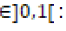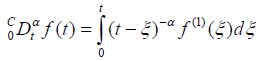(1)

Sizing of the variable ‘t’

For a thermal diffusion equation, the variable‘t‘represents the time in seconds. We will propose a simple procedure to construct the time fractional thermal diffusion equation. To do this, we replace the time ordinary derivative operator with the fractional as follows: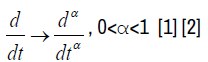(2)

Figure 1: Graphical representation of the temperature variation. If the time t varies between 0 and 1 and the position x between 0 and 2, when the order of the fractional derivative is 0.9.

We can see that (2) is not quite exact, from a physical point of view, because the derivative operator of time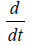has for dimension the inverse of the second s−1 and that of the operator of the fractional derivative of time is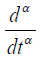. In order to be consistent with the dimensionality of time we introduce the new parameter σ with the following way: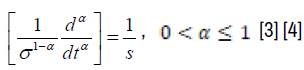(3)

where α is an arbitrary parameter which represents the order of the derivative. In the case where α = 1, expression (3) becomes an ordinary derived operator. In this way (3) is dimensionally consistent if and only if the new parameter has the dimension of time [σ] = s. Hence we have a simple procedure to construct fractional differential equations. It consists to replace in the following, the ordinary derivative in the ordinary differential equation by the fractional derivative operator: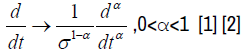(4)

Expression (4) is a time derivative in the usual sense, because its dimension is s−1 . The parameter σ (auxiliary parameter) represents the fractional time components in the system [3,4].

Expression of the fractional thermal diffusion equation

If t is the temporal variable and x the spatial variable, the classical thermal diffusion equation is written: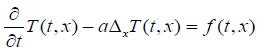(5)

• ‘a’ is the diffusivity coefficient

• T(t, x) is the temperature corresponding to the variables t and x

• f (t, x) is the second member of equation (1) for the variables t and x

• t> 0 and x belongs to a certain domain of space

From the previous paragraph the fractional thermal diffusion equation with respect to time is written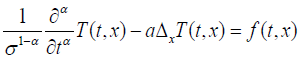In our case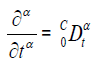Finally, the fractional thermal diffusion equation with respect to time is written: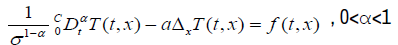(6)

Note:

The parameter α , which represents the order of the fractional derivative, can be related to the parameter σ, which characterizes the presence of fractional structures in the system. For the present case, referring to the principle taken in  and , (6) is written: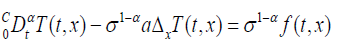And we take α =σ a ,(6) is written: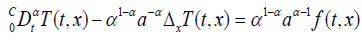(7)

Discretization and simulation

Discretization

For our discretization, it suffices to consider the variable x only on the real line because σ has no impact on the spatial variable: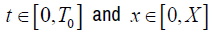On the segment [ 0,T0 ], we build a finite sequence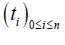such that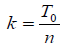and ti = ik - Similarly, on the segment [0, X ] , a finite sequence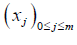such that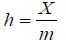and xj = jh

According to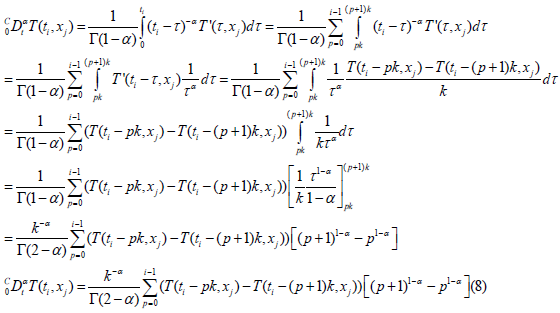This relation is valid for i ≠ 0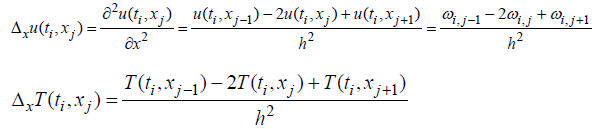(7) Is written :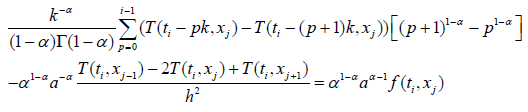More precisely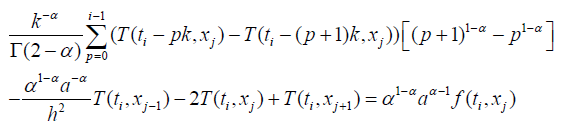Simulation

Make T0 =1; X0 = 2 , 0 <α <1 and we take as an initial condition T(0, x) = 0

for all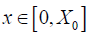The boundary condition is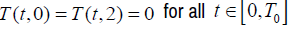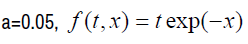(9) can still be written: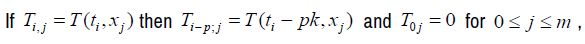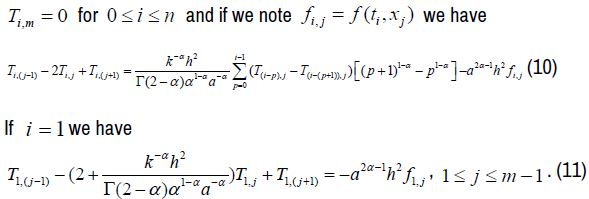If we vary j, we have m equations: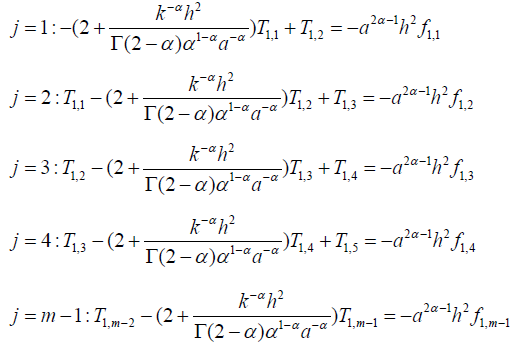We have the following matrix representation: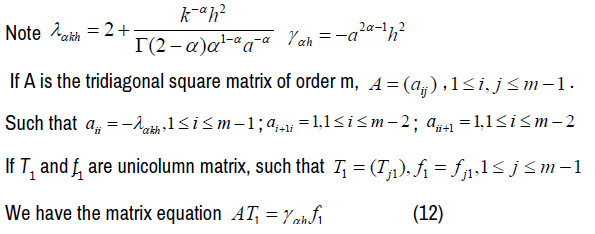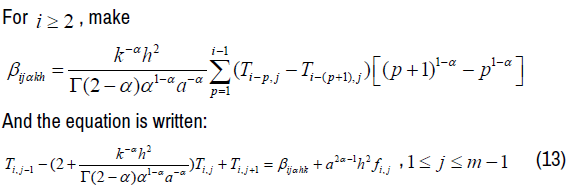And we have a matrix equation similar to the case i = 1. Thus, we have n matrix equations: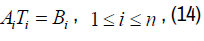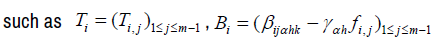Solving these equations, gives us the values of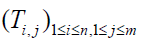From the above mentioned data, from MATLAB software, we get the following graphical representation:

#### Conclusion

The important point for this article is the introduction of the parameter ‘σ ‘ in the case of the fractional thermal diffusion equation with respect to time. But the problem that still arises is the identification of the expression of ‘σ’ in the general case, i.e: Is it possible to give a method to search ‘σ’ for all cases.

#### Journal Highlights

###### Citations: 1282

Journal of Applied & Computational Mathematics received 1282 citations as per Google Scholar report